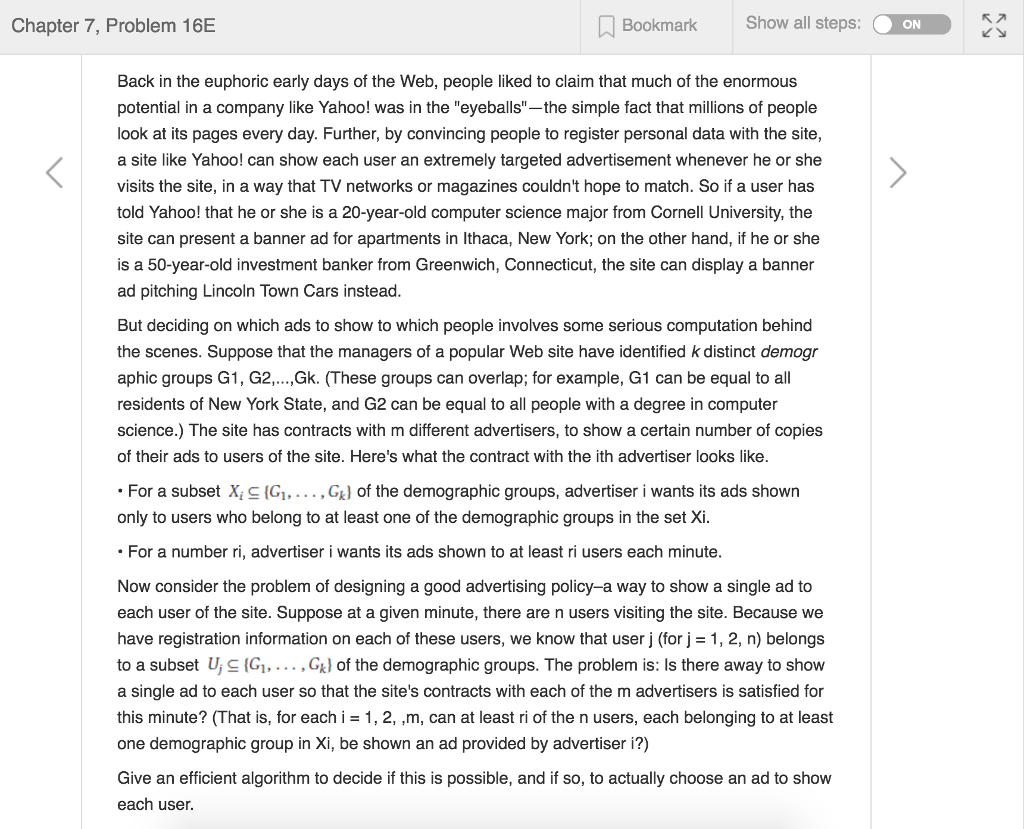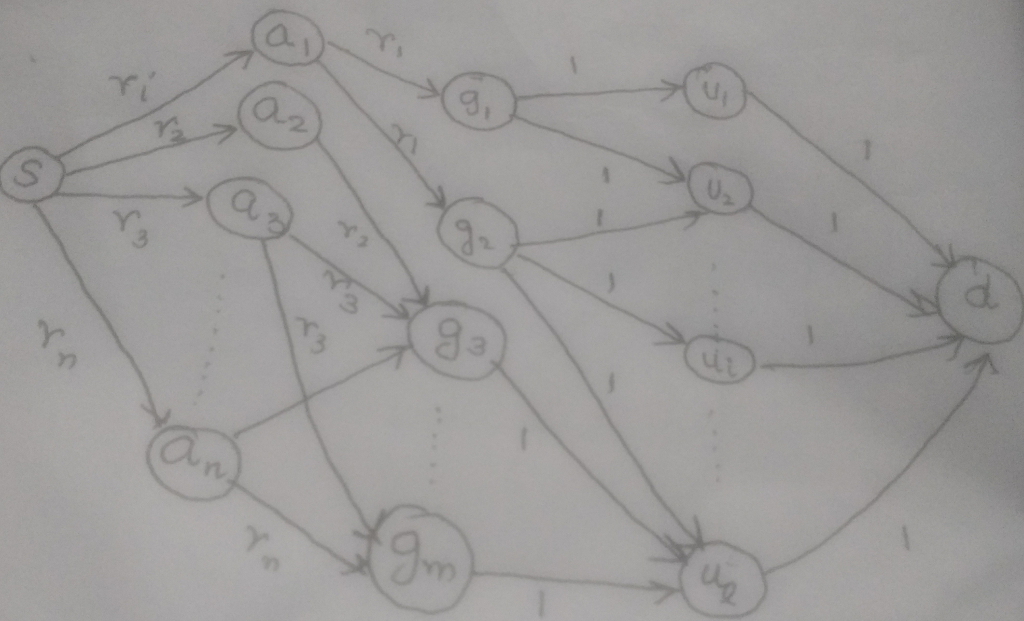# Answered! Back in the euphoric early days of the Web, people liked to claim that much of the enormous potential in a company…We will reduce the given problem to max-flow problem, which can then be solved using ford-fulkerson’s algorithm.

We add the following vertices to our graph:

Don't use plagiarized sources. Get Your Custom Essay on
Answered! Back in the euphoric early days of the Web, people liked to claim that much of the enormous potential in a company…
GET AN ESSAY WRITTEN FOR YOU FROM AS LOW AS \$13/PAGE
• For each User-i, we add a vertex ui.
• For each group-i, we add a vertex gi.
• Add a source vertex ‘s’ and sink vertex ‘d’.

Now, we add directed edges to the graph:

• For every advertiser, we add an edge from s to ai, with weight (capacity), ri. Meaning that we dont want more than ri ads to be shown for advertiser-i. ( The problem does not specify ri as sthe upper limit but since, we are trying to find out if there’s an assignment where at least ri ads can be served for the advertiser, servinging ri ads is sufficient and hence we are limiting this to ri)
• For every advertiser-i and group-j , we add an edge from ai to gj, with weight ri, if Gj Xi. This means that the flow of ads can only go into groups where advertiser wants to show ads. Again since the upper limit of ads for advertiser-i is ri, the upper limit of ads for advertiser-i shown to any group will have an upper limit of ri.
• For every user-i and group-j , we add an edge from gj to ui, with weight 1, if Gj Ui. This means that the flow of ads can move from groups to user only if the user belongs to that group. And since every user can be shown a single ad, we have set the capacity to 1.
• For every user-i, we add an edge from ui to d, with weight 1. To finalize our flow.

This is what the graph will look like:Now we solve the max-flow problem for this graph with source as s and sink as d. If the max flow is = say, R, for every advertiser-i, then at least ri users can be assigned to each advertiser-i.

Here’s the algorithm to assign users to the advertisers:

Create a graph G as per instructions above

Run ford-fulkerson’s algorithm on graph G. If the max flow is computed as R, a satisfactory assignment is possible

for each advertiser-i, flow between s and ai will be ri (as the max flow is computed as R)

for every and group-i and user-j:

for every and group-j:

f = flow(ai,gj) // flow from ai to gj as computed by the ford fulkerson’s algorithm

there will at least be f unassigned users such that flow(gj,uk) = 1, assign these f users to advertiser-i, and mark them assigned

——————————————————————–

Running time of the algorithm = running time of the ford-fulkerson algorithm = O(E*f).

Where f is the maximum outgoing flow at any edge which in this case is R, the sum of all ri’s, hence f =

E is the number of edges (= number of advertisers(say a) + number of users(say u) + sum of number of groups that each user is a part of + sum of number of groups that each advertiser is targeting) which is:

Hence the time complexity is: where a is the number of advertisers and u, the number of users.